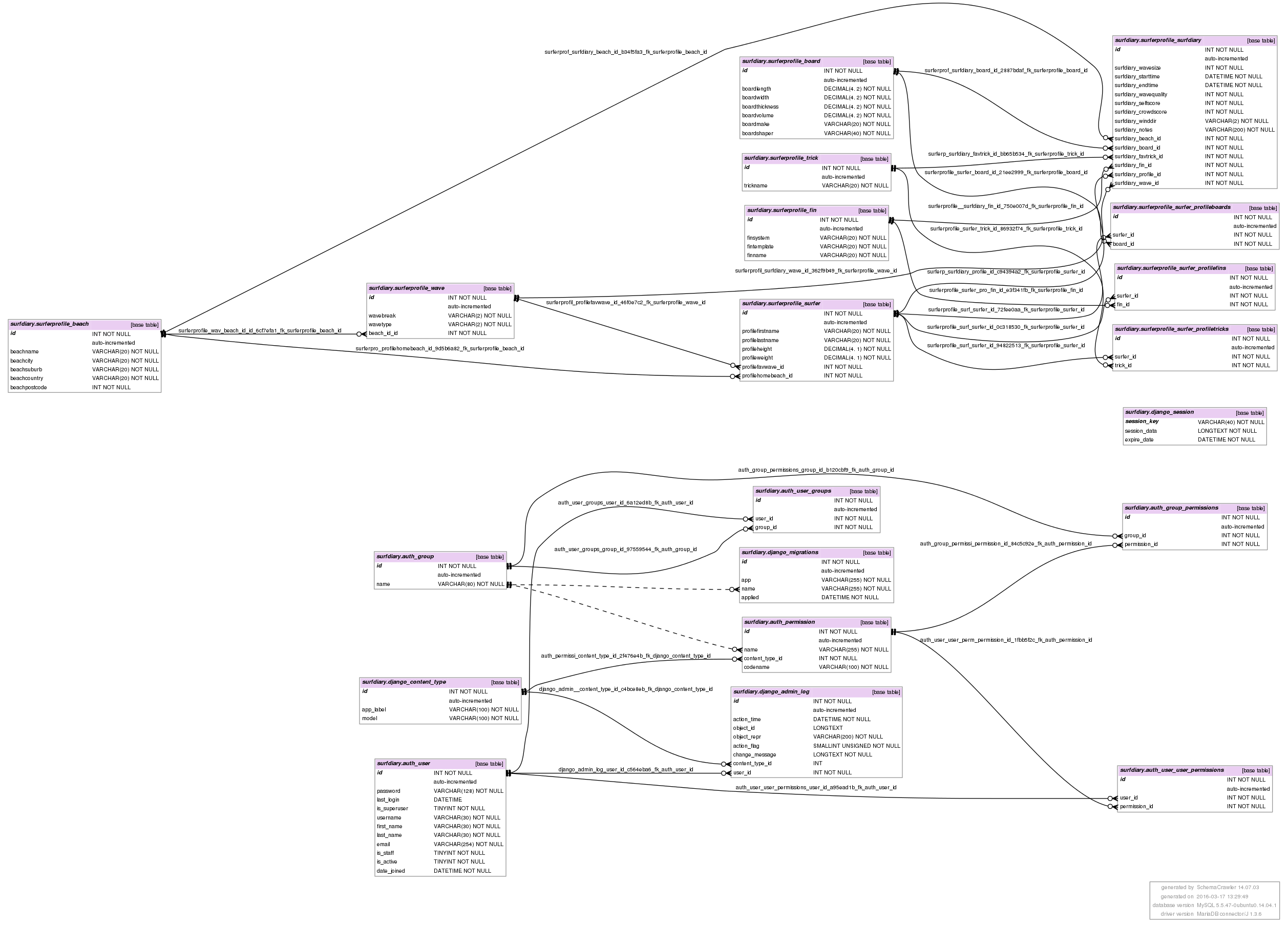## create model

Date: March 17th 2016
Last updated: March 17th 2016

A model is created in models.py and states exactly how the database should be designed. In this example I am going to add a full suite of tables which contain different field types and input restrictions. Useful tutorials worth looking at:

The steps:

``````# add to models.py
code below

# update settings.py (inside mysite directory, not the app)
#< snipped >
INSTALLED_APPS = [
'surferprofile.apps.SurferprofileConfig', #<==== added app
'django.contrib.admin',
'django.contrib.auth',
'django.contrib.contenttypes',
'django.contrib.sessions',
'django.contrib.messages',
'django.contrib.staticfiles',
]
#< snipped >

# makemigrations
python3 manage.py makemigrations surferprofile

# migrate
python3 manage.py migrate

# output from migrate command
#Operations to perform:
#  Apply all migrations: admin, auth, contenttypes, sessions, surferprofile
#Running migrations:
#  Rendering model states... DONE
#  Applying surferprofile.0001_initial... OK
``````

models.py
Things to note include ForeignKey and ManyToMany relationships,

``````from django.db import models

class Beach(models.Model):
beachname = models.CharField(max_length=20)
beachcity = models.CharField(max_length=20)
beachsuburb = models.CharField(max_length=20)
beachcountry = models.CharField(max_length=20)
beachpostcode = models.IntegerField()

class Wave(models.Model):
# break choice
POINTBREAK = 'PB'
BEACHBREAK = 'BB'
REEF = 'RF'
WAVES_CHOICE = ((POINTBREAK, 'Point break'), \
(BEACHBREAK, 'Beach break'), \
(REEF, 'Reef'),)
wavebreak = models.CharField(max_length=2, choices=WAVES_CHOICE,
default=BEACHBREAK)
# Wave type
CLOSEOUTS = 'CO'
CRUMBLY = 'CR'
REFORMS = 'RE'
TUBING = 'TU'
DOUBLEUPS = 'DU'
WAVE_TYPE = ((CLOSEOUTS, 'Closeouts'),
(CRUMBLY, 'Crumbly'),
(REFORMS, 'Reforming'),
(TUBING, 'Hollow tubing'),
(DOUBLEUPS, 'Double-ups'),)
wavetype = models.CharField(max_length=2, choices=WAVE_TYPE,
default=TUBING)
beach_id = models.ForeignKey(Beach, on_delete=models.CASCADE)

class Board(models.Model):
boardlength = models.DecimalField(max_digits=4,decimal_places=2)
boardwidth = models.DecimalField(max_digits=4,decimal_places=2)
boardthickness = models.DecimalField(max_digits=4,decimal_places=2)
boardvolume = models.DecimalField(max_digits=4,decimal_places=2)
boardmake = models.CharField(max_length=20)
boardshaper = models.CharField(max_length=40)

class Fin(models.Model):
finsystem = models.CharField(max_length=20)
fintemplate = models.CharField(max_length=20)
finname = models.CharField(max_length=20)

class Trick(models.Model):
trickname = models.CharField(max_length=20)

class Surfer(models.Model):
profilefirstname = models.CharField(max_length=20)
profilelastname = models.CharField(max_length=20)
profileheight = models.DecimalField(max_digits=4, decimal_places=1)
profileweight = models.DecimalField(max_digits=4, decimal_places=1)
profilehomebeach = models.ForeignKey(Beach, on_delete=models.CASCADE)
profilefavwave = models.ForeignKey(Wave, on_delete=models.CASCADE)
profilefins = models.ManyToManyField(Fin)
profiletricks = models.ManyToManyField(Trick)
profileboards = models.ManyToManyField(Board)

class SurfDiary(models.Model):
surfdiary_wavesize = models.IntegerField()
surfdiary_starttime = models.DateTimeField('start time')
surfdiary_endtime = models.DateTimeField('end time')

SCORE_CHOICES = zip( range(1,11), range(1,11) )
surfdiary_wavequality = models.IntegerField(choices=SCORE_CHOICES, blank=True)
surfdiary_selfscore = models.IntegerField(choices=SCORE_CHOICES, blank=True)
surfdiary_crowdscore = models.IntegerField(choices=SCORE_CHOICES, blank=True)

NONE = 'NO'
ONSHORE = 'ON'
OFFSHORE = 'OF'
CROSSSHORE = 'CR'
WINDDIR = ((NONE, 'No wind'),
(ONSHORE, 'On shore'),
(OFFSHORE, 'Off shore'),
(CROSSSHORE, 'Cross shore'),)
surfdiary_winddir = models.CharField(max_length=2, choices=WINDDIR)
surfdiary_notes = models.CharField(max_length=200)

surfdiary_profile = models.ForeignKey(Surfer, on_delete=models.CASCADE)
surfdiary_board = models.ForeignKey(Board, on_delete=models.CASCADE)
surfdiary_fin = models.ForeignKey(Fin, on_delete=models.CASCADE)
surfdiary_beach  = models.ForeignKey(Beach, on_delete=models.CASCADE)
surfdiary_wave  = models.ForeignKey(Wave, on_delete=models.CASCADE)
surfdiary_favtrick  = models.ForeignKey(Trick, on_delete=models.CASCADE)
``````

Schema
Note the separation of the default tables and the models just created. This is useful to visualise how each class gets mapped to a table in the database schema. Also note that all columns have been given NOT NULL by default, I will need to change this in the future.